Start typing, then use the up and down arrows to select an option from the list.# Naming Acids Example 1

Jules Bruno
151views
1
So for this example question, it says right the formula for each of the following compounds, we have hydro I otik acid Hydro's Olynyk acid and Hydrofluoric acid. All right, so we're going to say that hydro, we know that represents the H Plus ion involved. So we have HPE Plus for all of these, Since they're all binary acids, we know they all end with acid. What we look at is the base name. The base name tells us the nonmetal involved, so I owed means. We're dealing with iodine. It's in Group seven A. So what's minus one? Sillen means we're dealing with selenium. It's in Group six A. So it's two minus or minus two. And then floor means we're dealing with flooring, which is a group 78 So it is minus one now for the first one for a remember when the numbers and the charges are the same, they simply cancel out and you combine your elements together. So hydro I otik acid is just h I. And then also for C plus one minus one. The numbers are the same, so you just combine them together. So Hydrofluoric acid is HF for being Hydro's Olynyk acid. We have here different numbers in the charges. Remember, when the numbers are different, they crisscross. So one from here comes here to from here comes here. So that means Hydro's Olynyk acid is H two S e. If you don't quite remember these rules, make sure go back and take a look at our videos talking about writing ionic compounds. It's the same kind of principles behind this. We look at the ions and charges. If the numbers are the same, they simply cancel out if the numbers are different than they crisscross. Using this helps us to determine each one of these three binary acids. Now that we've done this example, move onto the next video where we take a look at a practice problem.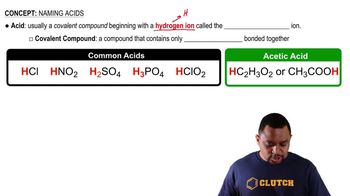02:15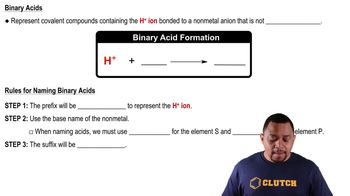02:34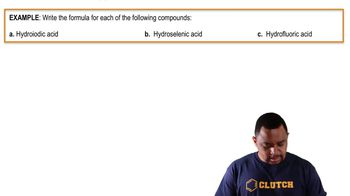02:21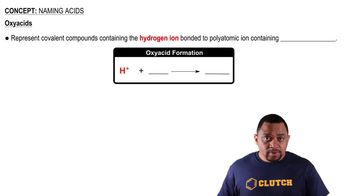01:18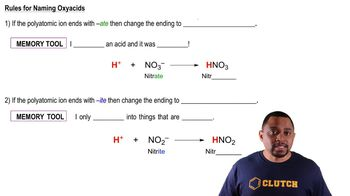02:49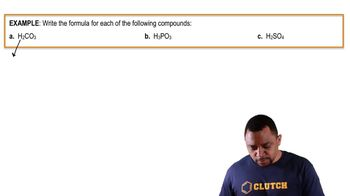02:42